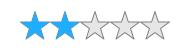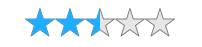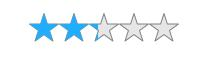# Rating Precisions in UWP Rating (SfRating)

10 May 20212 minutes to read

`Precision` specifies the level of accuracy in rating value. `SfRating` has three different precision types and they are:

• Standard
• Half
• Exact

## Rating the item completely

Rating item fills completely when pointer enters the rating item. In the following example, 2.3 has been set as the value and the rating item has been filled completely.

``````<input:SfRating ItemsCount="5" Value="2.3"
Precision="Standard" x:Name="rating"/>``````
``````rating.ItemsCount = 5;

rating.Value = 2.3;

rating.Precision = Syncfusion.UI.Xaml.Primitives.Precision.Standard;``````
``````rating.ItemsCount = 5

rating.Value = 2.3

rating.Precision = Syncfusion.UI.Xaml.Primitives.Precision.Standard``````## Rating the item partially

Rating item fills partially when pointer enters the rating item. In the following example, 2.3 has been set as the value and the rating item has been filled partially.

``````<input:SfRating ItemsCount="5" Value="2.3"
Precision="Half" x:Name="rating"/>``````
``````rating.ItemsCount = 5;

rating.Value = 2.3;

rating.Precision = Syncfusion.UI.Xaml.Primitives.Precision.Half;``````
``````rating.ItemsCount = 5

rating.Value = 2.3

rating.Precision = Syncfusion.UI.Xaml.Primitives.Precision.Half``````## Rating the item exactly

Rating item fills exactly when the pointer enters the rating item. In the following example, 2.3 has been set as the value and the rating item is filled to the exact value.

``````<input:SfRating ItemsCount="5" Value="2.3"
Precision="Exact" x:Name="rating"/>``````
``````rating.ItemsCount = 5;

rating.Value = 2.3;

rating.Precision = Syncfusion.UI.Xaml.Primitives.Precision.Exact;``````
``````rating.ItemsCount = 5

rating.Value = 2.3

rating.Precision = Syncfusion.UI.Xaml.Primitives.Precision.Exact``````